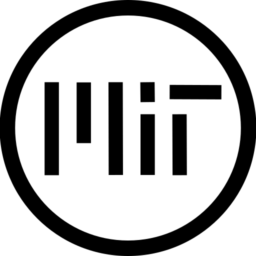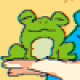# Stylized fire (3D)

Animated stylized 3D fire shader based on a Unity VFX guide by MinionsArt.

Similar to effects seen in games like Ryme.

You can assign different kinds of seamless noise textures to the shader to control the flame’s shape. In our demo repository, you’ll find 3 textures to play with.

See the demo scene StylizedFire.tscn in the GDQuest Godot shaders repository.

If you enjoy the effect, please leave a heart and star the repository!

``````// This shader is based on Minionsart's stylized fire
render_mode blend_mix;

uniform sampler2D noise_texture;

uniform float emission_intensity = 2.0;
uniform float time_scale = 3.0;
uniform vec2 texture_scale = vec2(1.0);
uniform float edge_softness = 0.1;

varying vec3 world_coord;
varying float world_x_dot;

void vertex() {
mat4 mat_world = mat4(normalize(CAMERA_MATRIX)*length(WORLD_MATRIX),normalize(CAMERA_MATRIX)*length(WORLD_MATRIX),normalize(CAMERA_MATRIX)*length(WORLD_MATRIX),WORLD_MATRIX);
mat_world = mat_world * mat4( vec4(cos(INSTANCE_CUSTOM.x),-sin(INSTANCE_CUSTOM.x), 0.0, 0.0), vec4(sin(INSTANCE_CUSTOM.x), cos(INSTANCE_CUSTOM.x), 0.0, 0.0),vec4(0.0, 0.0, 1.0, 0.0),vec4(0.0, 0.0, 0.0, 1.0));
MODELVIEW_MATRIX = INV_CAMERA_MATRIX * mat_world;

world_coord = (mat_world * vec4(VERTEX, 1.0)).rgb;
vec4 world_normal = (mat_world * vec4(NORMAL, 0.0));
world_x_dot =  abs(dot(normalize(world_normal.rgb), vec3(1.0,0.0,0.0)));
}

void fragment() {

vec2 time_based_pan = vec2(0.2, 1.0) * (- TIME * time_scale);
float noise_xy = texture(noise_texture, world_coord.xy * texture_scale + time_based_pan).r;
float noise_zy = texture(noise_texture, world_coord.zy * texture_scale + time_based_pan + vec2(0.7, 0.3)).r;

float noise = mix(noise_xy, noise_zy, clamp(world_x_dot, 0.0, 1.0));

ALBEDO = COLOR.rgb;
EMISSION = ALBEDO * emission_intensity;

float erosion_amount = (1.0 - COLOR.a);
float alpha = (noise * mask) - erosion_amount;

alpha = clamp(alpha, 0.0, 1.0);
ALPHA = smoothstep(0.0, edge_softness, alpha);
}``````
###### Tags
3d, cartoon, cel shading, fire, stylizedThe shader code and all code snippets in this post are under MIT license and can be used freely. Images and videos, and assets depicted in those, do not fall under this license. For more info, see our License terms.

## More from gdquest

### UCBC’s Stylized Light with Light Masking

Subscribe
Notify ofInline FeedbacksTotallyJustMagic
2 years ago

This is really cool! Is there a 2D version of this? can you make one?flytrap
2 months ago

I wrote one in the past, but it’s not necessarily the greatest looking. I may upload it if you want.gerardogc2378
8 months ago

For Godot 4.0.1

```void vertex() {
// Billboard code, taken directly from a spatial material
// create a spatial material, enable billboard with billboard keep scale, and then convert
// to shader material, and it will create a shader with this code.
mat4 mat_world = mat4(normalize(INV_VIEW_MATRIX)*length(MODEL_MATRIX),normalize(INV_VIEW_MATRIX)*length(MODEL_MATRIX),normalize(INV_VIEW_MATRIX)*length(MODEL_MATRIX), MODEL_MATRIX);
mat_world = mat_world * mat4( vec4(cos(INSTANCE_CUSTOM.x),-sin(INSTANCE_CUSTOM.x), 0.0, 0.0), vec4(sin(INSTANCE_CUSTOM.x), cos(INSTANCE_CUSTOM.x), 0.0, 0.0),vec4(0.0, 0.0, 1.0, 0.0),vec4(0.0, 0.0, 0.0, 1.0));
MODELVIEW_MATRIX = VIEW_MATRIX * mat_world;

// We map the coordinates on the vertical planes xy and zy
// we also calculate how to blend between the two based on where the world space normal
// is pointing.
world_coord = (mat_world * vec4(VERTEX, 1.0)).rgb;
vec4 world_normal = (mat_world * vec4(NORMAL, 0.0));
world_x_dot =  abs(dot(normalize(world_normal.rgb), vec3(1.0,0.0,0.0)));
}
```